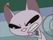cancel
Showing results for
Did you mean:Frequent Visitor

## dim_Range? One set of time ranges that classify multiple different columns

I have a ton of different duration columns in decimal number format.

I would like to be able to put them all into ranges.

EX:

each if [xduration] <= .25 then "00:01-00:15"

else if [xduration] <= .5 then "00:16-00:30"
else if [xduration] <= .75 then "00:31-00:45"
else if [xduration] <= 1 then "00:46-01:00"
etc.

What is the easiest way to implement this for numerous columns that will still be efficient during refresh?

4 REPLIES 4Responsive Resident

You can use a custom function in Power Query to apply this logic to multiple columns at once. Here's how you can do it:

1. In the Power Query Editor, click on the "View" tab and select "Advanced Editor".

2. In the Advanced Editor, paste the following code:

let
Source = <Your_Source_Table>,
durationColumns = List.Select(Table.ColumnNames(Source), each Text.StartsWith(_, "xduration")),
toRange = (duration as number) =>
if duration <= 0.25 then "00:01-00:15"
else if duration <= 0.5 then "00:16-00:30"
else if duration <= 0.75 then "00:31-00:45"
else if duration <= 1 then "00:46-01:00"
else "Other",
toRangeTable = (column as text) =>
let
columnRange = Table.AddColumn(Source, column & "_Range", each toRange([column])),
columnSelected = Table.SelectColumns(columnRange, column, column & "_Range")
in
columnSelected,
outputTable = List.Accumulate(durationColumns, Source, (t, c) => Table.Combine({t, toRangeTable(c)}))
in
outputTable

1. Replace <Your_Source_Table> in the code above with the name of your source table.

2. Click on the "Done" button to close the Advanced Editor.

3. The code above will create a custom function called "toRange" that maps the decimal number durations to the desired ranges. It also creates another custom function called "toRangeTable" that adds a new column to the source table with the range values.

4. Finally, the code uses the "List.Accumulate" function to apply the "toRangeTable" function to all columns that start with "xduration", and then combine the resulting tables into a single output table.

By using this custom function, you can easily apply the range logic to multiple duration columns at once, and the refresh performance should still be efficient.Frequent Visitor

What's the easiest way to use custom column names instead? They don't all start with xduration, that was just written as an example.

Edit : Did a test on a few columns by changing xduration to bin and get this error :
"Expression.Error: We cannot convert the value "bin_on_scene_duratio..." to type Number.
Details:
Value=bin_on_scene_duration_Range
Type=[Type]"Memorable Member

Hello, @Cheywork91 try this on your data. If you need to modify all columns in the table then duration/other columns selection steps are not necessary - just Table.ToColumns the whole table. Otherwise modify duration_column_names manually.

``````let
dt = Table.FromRows(Json.Document(Binary.Decompress(Binary.FromText("i45WKjFU0lEy0IOSxkqxOkAxIzAPQhpYQMRMwTwIaWQEETMH86CkKUTM0hLMhVIWQN2xAA==", BinaryEncoding.Base64), Compression.Deflate)), let _t = ((type nullable text) meta [Serialized.Text = true]) in type table [c1 = _t, c2 = _t, c3 = _t]),
data_table = Table.TransformColumnTypes(dt,{{"c3", type number}, {"c2", type number}}),
lt = Table.FromRows(Json.Document(Binary.Decompress(Binary.FromText("LcpBDgAQDETRu3SNzNCSuIq4/zUU3fzFy19LUKpJEmCC2UuTnS6Hsl9tCB3B7c36Z35TXznh6z4=", BinaryEncoding.Base64), Compression.Deflate)), let _t = ((type nullable text) meta [Serialized.Text = true]) in type table [d = _t, range = _t]),
lookup_table = Table.Sort( Table.TransformColumnTypes(lt,{{"d", type number}}), "d" ),
lookup_d = List.Buffer( lookup_table[d] ),
lookup_range = List.Buffer( lookup_table[range] ),
f = (x as list) =>
List.Transform(
x,
(w) =>
let
a = List.PositionOf( lookup_d, w, Occurrence.First, (a, b) => a >= b )
in try lookup_range{a} otherwise null
),
duration_column_names = {"c2", "c3"},
other_column_names = List.Difference( Table.ColumnNames( data_table ), duration_column_names ),
duration_columns =
List.Transform(
Table.ToColumns(
Table.SelectColumns( data_table, duration_column_names )
),
each f (_)
),
other_columns = Table.ToColumns( Table.SelectColumns(data_table, other_column_names) ),
final_table = Table.FromColumns(other_columns & duration_columns, other_column_names & duration_column_names)
in
final_table``````Frequent Visitor

This looks promising, but I'm not comfortable importing an unknown JSON.

Thanks anyways